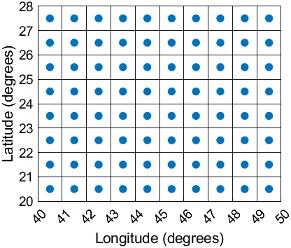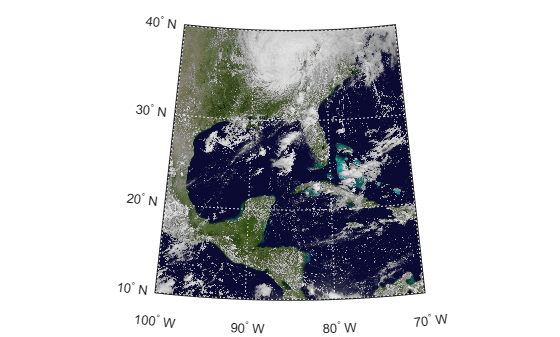GeographicCellsReference

Reference raster cells to geographic coordinates

Description

A geographic cells raster reference object contains information that describes the relationship between a geographic coordinate system and an intrinsic coordinate system that is anchored to the columns and rows of a 2-D spatially referenced raster grid or image of cells.

In order to reference a raster of cells to geographic coordinates by using a GeographicCellsReference object, the raster must be sampled regularly in latitude and longitude, and its columns and rows must be aligned with meridians and parallels, respectively. The sample spacing from row to row need not equal the sample spacing from column to column.

This image illustrates a 8-by-10 geographic raster of cells. The raster cells have an extent of 1 degree in latitude and longitude, cover longitude values in the range [40,50] in degrees, and cover latitude values in the range [20,28] in degrees. The boundary of the raster of cells is made up of the outermost boundaries of the outermost cells.To reference a raster of postings to geographic coordinates, use a GeographicPostingsReference object instead.

Creation

You can use these functions to create GeographicCellsReference objects:

• readgeoraster — Import a raster data file as an array and a reference object.

• wmsread — Import a raster map from a Web Map Service (WMS) server as an array and a reference object.

• georefcells — Create a geographic raster reference object by specifying the latitude and longitude limits of the raster and either the size of the raster or the extent of the cells.

• georasterref — Convert a world file to a geographic raster reference object.

• refmatToGeoRasterReference — Convert a referencing matrix to a geographic raster reference object.

• refvecToGeoRasterReference — Convert a referencing vector to a geographic raster reference object.

Properties

expand all

Latitude limits of the geographic quadrangle bounding the georeferenced raster, specified as a two-element vector of the form [southern_limit northern_limit].

Example: [-90 90]

Data Types: double

Longitude limits of the geographic quadrangle bounding the georeferenced raster, specified as a two-element vector of the form [western_limit eastern_limit].

Example: [-100 180]

Data Types: double

Number of rows and columns of the raster or image associated with the referencing object, specified as a two-element vector, [m n], where m represents the number of rows and n the number of columns. For convenience, you can assign a size vector having more than two elements. This enables assignments like R.RasterSize = size(RGB), where RGB is m-by-n-by-3. In cases like this, the object stores only the first two elements of the size vector and ignores the higher (nonspatial) dimensions.

Example: [200 300]

Data Types: double

Geometric nature of the raster, specified as 'cells'. The value 'cells' indicates that the raster comprises a grid of quadrangular cells, and is bounded on all sides by cell edges. For an m-by-n raster, points with an intrinsic x-coordinate of 1 or n or an intrinsic y-coordinate of 1 or m fall within the raster, not on its edges.

Data Types: char

Unit of measurement used for angle-valued properties, specified as 'degree'.

Cannot be set.

Data Types: char

Edge from which column indexing starts, specified as 'south' or 'north'.

Example: 'south'

Data Types: char

Edge from which row indexing starts, specified as 'west' or 'east'.

Example: 'east'

Data Types: char

Extent in latitude of individual cells, specified as a positive numeric scalar. Distance, in units of latitude, between the northern and southern limits of a single raster cell. The value is the same for all cells in the raster.

Example: 2.5

Data Types: double

Extent in longitude of individual cells, specified as a positive numeric scalar. Distance, in units of longitude, between the western and eastern limits of a single raster cell. The value is always positive, and is the same for all cells in the raster.

Example: 2.5

Data Types: double

Latitude extent ("height") of the quadrangle covered by the raster, specified as a positive numeric scalar.

Example: 2

Data Types: double

Longitude extent ("width") of the quadrangle covered by the raster, specified as a positive numeric scalar.

Data Types: double

Raster limits in intrinsic x coordinates, specified as a two-element row vector of positive integers, [xMin xMax]. For an m-by-n raster, XIntrinsicLimits equals [0.5, m+0.5], because the RasterInterpretation is 'cells'.

Example: [0.5 2.5]

Data Types: double

Raster limits in intrinsic y coordinates, specified as a two-element row vector of positive integers, [yMin yMax]. For an m-by-n raster, YIntrinsicLimits equals [0.5, n+0.5], because the RasterInterpretation is 'cells'.

Data Types: double

Type of coordinate system to which the image or raster is referenced, specified as 'geographic'.

Data Types: char

Geographic coordinate reference system (CRS), specified as a geocrs object. A geographic CRS consists of a datum (including its ellipsoid), prime meridian, and angular unit of measurement.

Object Functions

 contains Determine if geographic or map raster contains points geographicGrid Geographic coordinates of raster elements geographicToDiscrete Transform geographic to discrete coordinates geographicToIntrinsic Transform geographic to intrinsic coordinates intrinsicToGeographic Transform intrinsic to geographic coordinates intrinsicXToLongitude Convert from intrinsic x to longitude coordinates intrinsicYToLatitude Convert from intrinsic y to latitude coordinates latitudeToIntrinsicY Convert from latitude to intrinsic y coordinates longitudeToIntrinsicX Convert from longitude to intrinsic x coordinates sizesMatch Determine if geographic or map raster object and image or raster are size-compatible worldFileMatrix Return world file parameters for transformation

Examples

collapse all

Import a GeoTIFF image as an array and a GeographicCellsReference object by using the readgeoraster function.

View the properties of the GeographicCellsReference object.

R
R =
GeographicCellsReference with properties:

LatitudeLimits: [10 40]
LongitudeLimits: [-100 -70]
RasterSize: [1024 1024]
RasterInterpretation: 'cells'
ColumnsStartFrom: 'north'
RowsStartFrom: 'west'
CellExtentInLatitude: 0.029296875
CellExtentInLongitude: 0.029296875
RasterExtentInLatitude: 30
RasterExtentInLongitude: 30
XIntrinsicLimits: [0.5 1024.5]
YIntrinsicLimits: [0.5 1024.5]
CoordinateSystemType: 'geographic'
GeographicCRS: [1x1 geocrs]
AngleUnit: 'degree'

Create a world map with limits that match the limits of the reference object. Then, display the image.

worldmap(Z,R)
geoshow(Z,R)